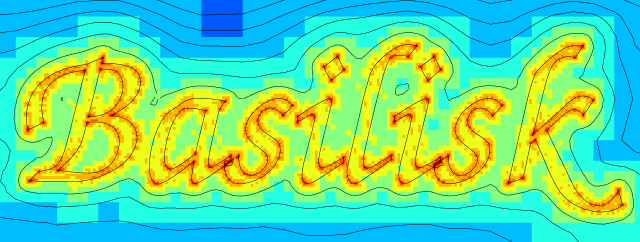# Computation of a levelset field from a contour

This test case illustrates how to import a contour, represented using a vector graphics file format, here an encapsulated Postscript file and represent it within Basilisk as a distance (i.e. levelset) field.

We will also use this distance function to compute a VOF representation of the contour, and test the tag() function.

#include "utils.h"
#include "distance.h"
#include "fractions.h"
#include "tag.h"
#include "view.h"

int main()
{

The .eps file was created using inkscape (or any other vector graphics editor). The .gnu file is obtained from the .eps file using

pstoedit -f gnuplot -flat 0.1 basilisk.eps basilisk.gnu

This gives the following curve

set term @SVG size 640,240
set size ratio -1
plot 'basilisk.gnu' w l t ''(script)

The pairs of coordinates defining the (oriented) segments are then read using:

  coord * p = input_xy (fopen ("basilisk.gnu", "r"));

We can optionally add noise to the representation, to check the robustness of the reconstruction for self-intersecting, non-closed curves.

#if 0
double amp = .0;
coord * i = p;
while (i->x != nodata) {
i->x += amp*noise(), i->y += amp*noise();
printf ("%g %g\n", i->x, i->y);
i++;
i->x += amp*noise(), i->y += amp*noise();
printf ("%g %g\n\n", i->x, i->y);
i++;
}
fflush (stdout);
#endif

init_grid (8);
size (105);
origin (-5, -5);

We initialise the distance field d and refine the mesh according to the error on this field.

  scalar d[];
distance (d, p);
while (adapt_wavelet ({d}, (double[]){1e-2}, 12).nf);

We tag each letter (counting the dots on the i’s).Tagged regions.

  scalar tt[];
foreach()
tt[] = d[] < 0;
int n = tag (tt);
output_ppm (tt, file = "tag.png", n = 512, box = {{-5,-5},{100,30}},
map = randomap, min = 0, max = n);
assert (n == 8);

We initialise a vertex distance field by interpolating the centered distance field, and use this to compute VOF fractions and facets.

  vertex scalar phi[];
scalar f[];
foreach_vertex()
phi[] = (d[] + d[-1] + d[0,-1] + d[-1,-1])/4.;
face vector s[];
fractions (phi, f, s);
output_facets (f, stderr, s);

Here we compute the number of segments intersected by the neighborhood (a sphere of diameter 3\Delta) of each cell.

  scalar nt[], surface = d.surface;
foreach() {
nt[] = 0;
if (surface[]) {
coord ** p = (coord **) double_to_pointer (surface[]);
while (*p)
nt[]++, p++;
}
}

We display the resulting curves and meshes.

  view (fov = 6.98459, tx = -0.444569, ty = -0.137936,
width = 640, height = 242);
for (double val = -20; val <= 20; val += 2.)
isoline ("phi", val, lw = 0.5);
squares ("level", min = 2, max = 12);
save ("mesh.png");Adaptive mesh and isolines of distance function.

Note that the input curve is self-intersecting. The algorithm used to compute the distance function is robust but gives the artefact illustrated below.

  view (fov = 1.64446, tx = -0.309322, ty = -0.0840683,
width = 449, height = 303);
draw_vof ("f", "s");
save ("self.png");The input curve represented through its VOF discretisation.](basil

}